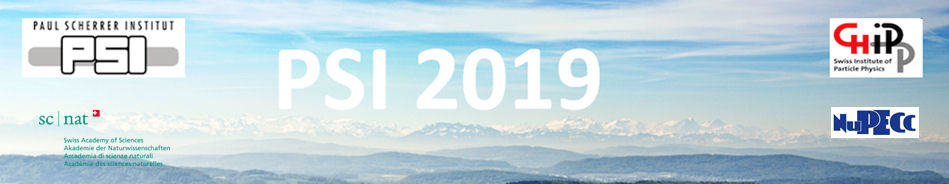#Physics of fundamental Symmetries and Interactions - PSI2019

Oct 20 – 25, 2019
PSI
Europe/Zurich timezone

## Rare pion decay studies in the PEN experiment

Oct 23, 2019, 9:00 AM
30m
WHGA/001 - Auditorium (PSI)

Invited Talk

### Speaker

Dinko Pocanic (University of Virginia)

### Description

Relatively simple dynamics, and extremely well controlled radiative and loop corrections, ensure that pion and muon decays are described with unprecedented precision within the Standard Model, typically with relative uncertainties of $\sim 10^{-4}$ or lower. This theoretical precision makes the $\pi$ and $\mu$ decays suitable as a sensitive means of testing the underlying symmetries, especially the universality of weak fermion and quark couplings. Of particular interest is the ratio $R_{e/\mu}^\pi = {\Gamma(\pi \to e\bar{\nu}_e(\gamma))}/{\Gamma(\pi \to \mu\bar{\nu}_{\mu}(\gamma))}$ which provides the current best limit on the universality of $W$ coupling to the first two lepton generations, i.e., $(e,\nu_e)$ and $(\mu,\nu_{\mu})$, respectively, with broad implications, including in the neutrino sector. The PEN collaboration is at the threshold of obtaining new results for $R_{e/\mu}^\pi$ at sub-$10^{-3}$ relative precision from measurements completed at PSI several years ago. We discuss the status of the PEN data analysis for several rare decay channels, the expected uncertainty limits, as well as complementarity with results of high-energy studies.

### Primary author

Dinko Pocanic (University of Virginia)